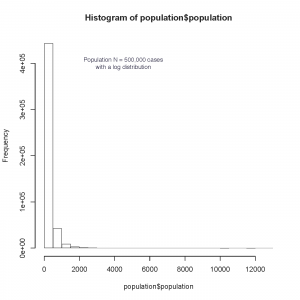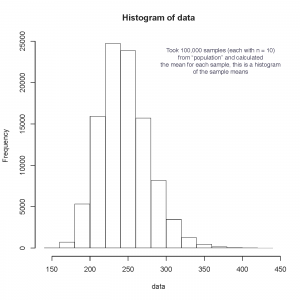# Law of large numbers – central limit theorem

I just came up with a simple example of the central limit theorem after teaching this concept to the DPT research methods course and getting a post class question. In class I had used a normal distribution of HRs from about 270,000 subjects (a large dataset of resting HR and BP I have of people getting pre-employment testing). We took this sample to be our population for example purposes. The initial purpose was to demonstrate the law of large numbers. That as we randomly drew larger samples from this population of 274,000 our sample mean and sample distribution started to be the same as the population mean and population distribution. And if the underlying population distribution is not normal, then that is the distribution that is obtained with a large sample. So if there is a right skew to a population distribution then as samples get larger then we expect that sample to have a right skew. That is the law of large numbers.

Central limit theorem (sometimes confused with the law of large numbers) is about the mean and distribution of “sample means.” You should think of it as the mean of means (though it also applies to the sum). If we have population with a “non normal” distribution, and we sample from it many times all with the same size sample, and we take the means of those samples, and then we make a histogram of those “sample means” then we end up with a normal distribution – no matter what the underlying population distribution might be.

To demonstrate I wrote the following R code that first creates a population with a log distribution and 500,000 “subjects.” There is then a function samples from that population with parameters you can vary (number of samples from the population, and number of subjects / sample), and creates a new data vector of the “sample means.”  The only R add on package used is: dplyr

The code is attached, and below is the underlying population distribution as well as the sample means distribution. This is the central limit theorem at work.

CLT_code